• 2021-05-18 13:26:35

综合性实践学习选题

总体要求：

一般每题要求四个函数，输入函数可以从in.dat中读数据，也可用rand()函数自行产生，输出函数完成把结果输出到屏幕或文件的任务，核心处理函数完成题目规定的要求，main()函数要求以尽可能友好、对程序使用安全的界面完成调用其它函数的任务。

一．排序求平均值(包括将数拆散，求奇偶数、求最大最小值)。

例如：5591是素数，则该数满足条件存入数组b中，且个数cnt=cnt+1。

9812是非素数，则该数不满足条件忽略。

4．请编制程序，读取200个整数至数组xx中，不计数组xx中值最大和最小的数(若有重复值，则都不计)，再求出最大数max及最大数的个数cnt1、最小数min及最小数的个数cnt2和所剩下200-cnt1-cnt2个数的算术平均值pj (保留2位小数)。输出结果max,cnt1,min,cnt2,pj。要求用三个以上的函数完成。

例：处理前 9012 5099 6012 7025 8088

处理后 5099 8088 7025 6012 9012

更多相关内容
• 大一写的结课作业，代码用到了文件、数组、结构体、函数，包括增删改查和成绩排名等功能，欢迎大家下载使用。
• ## 大一C语言大作业--扫雷雷

万次阅读 多人点赞 2020-07-13 14:35:33
说实话，其实当时选题的时候就有点偷懒了，学校给的那15个样例大作业我不怎么感兴趣（因为说白了就是过去oi里普及-的模拟/高精度算法），本来自己想完善一下高中写的那个人机五子棋的，emmmm但算法思路一直想不出...

## 写在前面

这篇文章并没有什么特别的地方，可以说是很烂大街的一个选题了。写的目的呢只是想把大作业留个纪念（说不定n年后再看这段代码会觉得自己写了一坨屎233）

说实话，其实当时选题的时候就有点偷懒了，学校给的那15个样例大作业我不怎么感兴趣（因为说白了就是过去oi里普及-的大模拟/高精度算法），本来自己想完善一下高中写的那个人机五子棋的，emmmm但算法思路一直想不出一个更好的（现在看来高中写的那个东西太shit了233），所以我再次选择了扫雷这个经典大作业作为我第一个用C++写的超过200行的程序。

## 以下正文

适用参考人群
大一非计算机类专业本科生（比如俺）的C/C++程序设计大作业，对编程感兴趣的初心者。985大学的同学就不用看了233，估计我这个东西到不了你们老师的要求，我觉得好多985的大作业挺顶的（比如整个元气骑士雷课堂啥的）
为什么扫雷小游戏适合大作业呢
因为通常来说，C语言程序设计的公共基础课，一般就是学变量、数据类型、字符串、顺序选择循环结构、递归、两大经典排序（冒泡和选择）、指针、结构体和文件操作函数。我这个方案除了指针，其他方面全部都涉及到了（当然数组遍历也可以用指针实现），对于入门来说应该算是比较理想的，难度不是很高，而且一般能实现的话老师的给分基本上也比较理想。
这个方案唯一需要自己额外去学的，只有一些基础的Windows.h头文件的内容（本方案中主要是用了鼠标操作和彩色字符输出的相关函数）

功能实现
实现经典Windows扫雷游戏功能。
能够实现的具体功能如下：
1） 游戏有四种模式可供选择
初级：8x8 10个雷
中级：16x16 40个雷
高级：30x16 99个雷
自定义：棋盘大小和地雷数量由用户输入，按照要求生成指定的棋盘和布雷。
2） 点击鼠标左键翻开格子。
3） 鼠标右键可对未翻开过的格子（‘#’）进行插旗子/拔旗子（程序中旗子用‘@’代替）的操作。
4） 可以实时显示游戏进行时间和剩余雷数
5） 游戏结束后通过文件操作函数更新最快用时排名表（写入data.txt），该排名表可反复调用修改写入新的数据。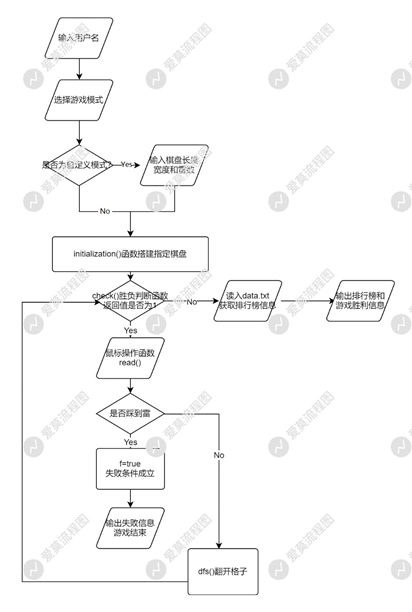## 搭建棋盘

使用到的知识：swtich选择，数组、结构体数组。

首先，先给用户起一个名字。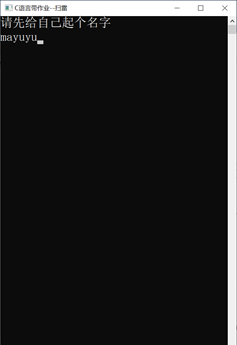然后，输入数字以选择游戏模式，如果是自定义模式（输入4时）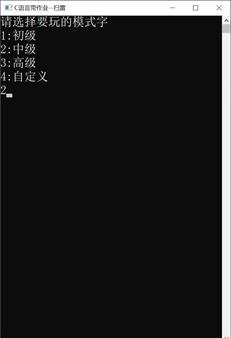然后利用随机函数+一个while循环枚举每个雷的横纵坐标，并布雷，接着统计每个格子周围的雷数。
在统计无雷格子周围地雷数时，定义：
int dx={0,0,1,-1,1,-1,1,-1};
int dy={1,-1,0,0,-1,1,1,-1};
（老oi做法了）
两个方向数组，表示八个不同的扩展方向（南、北、东、西、东南、西北、东北、西南），这样做的好处是程序编写起来简洁明了，每个格子周围八个位置只需要一个循环语句即可完成遍历。

void initialization(){  //初始化游戏界面
int len,width,x1=0,y1=0;
//houtput=GetStdHandle(STD_OUTPUT_HANDLE);
SetConsoleTitleA("C语言带作业--扫雷");
cout<<"请先给自己起个名字";
gotoxy(0,1);
gets(id);
gotoxy(0,0);
cout<<"请选择要玩的模式\n";
cout<<"1:初级\n";
cout<<"2:中级\n";
cout<<"3:高级\n";
cout<<"4:自定义\n";
gotoxy(0,5);
cin>>mode;
for (int i=1; i<=10; i++){
gotoxy(0,i-1);
cout<<"                                ";
}
s=clock();
switch (mode){
case 1:{n=8; m=8; t=10; len=28; width=28; break;}  //初级： 8x8 10个雷
case 2:{n=16; m=16; t=40; len=36; width=36; break;} //中级：16x16 40个雷
case 3:{n=30; m=16; t=99; len=50; width=36; break;} //高级： 30x16 99个雷
case 4:{gotoxy(0,0);
for (int i=1; i<=6; i++){
cout<<"                 \n";
}
gotoxy(0,0);
scanf("%d%d%d",&n,&m,&t); len=n+20; width=m+20;
break;
}
//自定义棋盘大小和雷数
}
WORD wr1 =0x0f;//定义颜色属性；第一位为背景色，第二位为前景色
SetConsoleTextAttribute(houtput,wr1);
srand(time(NULL)); //防伪随机
int ok;
for (int i=1; i<=t; i++){  //使用随机函数rand()%(y-x)+x，枚举棋盘范围内的所有地雷的随机位置坐标
do{
x1=rand()%(n-1)+1; y1=rand()%(m-1)+1; ok=1;
if (mapp[x1][y1].occupy){
mapp[x1][y1].occupy=false; mapp[x1][y1].ch='*'; ok=0;
}
}while(ok);
}
gotoxy(0,0);
cout<<"                              ";
SetConsoleTextAttribute(houtput,0x04);
gotoxy(1,0); cout<<t<<"あと"; //gotoxy(n/2-1,0); cout<<;
for (int i=1; i<=5; i++){
gotoxy(0,i); putchar(' ');
}
SetConsoleTextAttribute(houtput,0x0f);
for (int i=1; i<=n; i++){
for (int j=1; j<=m; j++){
gotoxy(i,j); putchar('#');
for (int k=0; k<8; k++)
if (mapp[i+dx[k]][j+dy[k]].ch=='*' && mapp[i][j].occupy)
mapp[i][j].num++;
}
}
}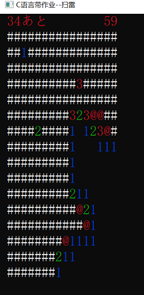## 游戏进行时

使用到的算法：鼠标操作函数、循环、选择、简单递归搜索

用一个while（1）循环来包装你整个游戏的操作过程（鼠标事件输入啊，输出字符啊、胜负判断的什么的）

鼠标事件分为点击左键和点击右键
点击左键时开雷
根据扫雷规则，如果翻开的格子不是雷，那么按照以下规则翻开格子：如果此处提示该处周围格地雷数大于0，则仅显示该地雷数（彩色输出），如果为0，则需继续翻开周围连续的格子以扩展翻开格子的区域，直至遇到边界全是大于0的数字为止，此外，提示雷数为0时应该输出空格而不是数字0。
因此我使用了深度优先遍历，利用之前定义的方向数组dx,dy去扩展结点，扩展的过程中更新格子的访问状态mapp[x][y].vis和mapp[x][y].occupy（该处格子的实际扩展情况），直至扩展到边界为止。

void dfs(int x,int y){
int x1,y1;
gotoxy(x,y); write(mapp[x][y].num); mapp[x][y].occupy=false; mapp[x][y].vis=true;
if (mapp[x][y].num>0) return;  //扩展到数字时停止搜索
for (int i=0; i<7; i++){
x1=x+dx[i]; y1=y+dy[i];
if (!mapp[x1][y1].vis &&  x1>0 && y1>0 && x1<=n && y1<=m){
dfs(x1,y1);
}
}
}


鼠标左键仅完成翻开格子的动作，如果翻开的格子为雷，则f=true（游戏失败条件成立），输出游戏失败信息，结束循环，游戏结束。如果翻开的格子不是雷，则调用dfs()按照扫雷规则展开格子。展开结束后，利用check()判断游戏是否结束，即是否所有的雷都被找出来了（胜利条件）
check()函数中先判断踩雷条件f是否成立，如果成立就直接退出，否则遍历整个棋盘，由于事先将已翻过的格子和布雷的位置mapp[x][y].occupy的信息更新为false状态，所以仅需找是否依旧存在mapp[x][y].occupy==true的格子存在，如果没有则游戏结束。

int check(){   //判断是否游戏结束
if (f) return 0; //踩了雷，bad end
for (int i=1; i<=n; i++)
for (int j=1; j<=m; j++)
if (mapp[i][j].occupy)
return 1;
return 0;
}


点击右键时插旗/拔旗
根据扫雷插旗的规则，已翻开的格子上不能插旗；对于未翻开的格子，可以点击鼠标右键进行插旗，如果格子处于插上旗子的状态下，点击左键就不能再翻开了，这里使用mapp[x][y].flag特判此未翻开格子是否有插上旗子，同时定义mapp[x][y].vis为格子是否为假翻开状态（因为正常情况下点击左键是可以翻开格子的），最后可以再次点击右键拔掉已插上的旗子。

	if (mouseRecord.Event.MouseEvent.dwButtonState == RIGHTMOST_BUTTON_PRESSED) //按下右键时插旗子/拔旗子
{
if (!mapp[(int)pos.X][(int)pos.Y].vis && (int)pos.X>0 && (int)pos.X<=n && (int)pos.Y>0 && (int)pos.Y<=m){
//该位置是合法坐标且未被访问过，实际演示中表现为只能在#上插旗子
gotoxy((int)pos.X,(int)pos.Y);
if (mapp[(int)pos.X][(int)pos.Y].flag){
write(d);
t++;
}
else{
write(-d); t--;
}
SetConsoleTextAttribute(houtput,0x04);
gotoxy(1,0); cout<<"  ";
gotoxy(1,0); cout<<t;
mapp[(int)pos.X][(int)pos.Y].flag=!mapp[(int)pos.X][(int)pos.Y].flag;
}
}


鼠标不点击时更新计时器。
（由于我不会多线程，所以计时器用一种原始方法实现的）

e=clock(); //游戏终止时刻
duration=(int)(e-s) / CLOCKS_PER_SEC; //游戏进行时间
SetConsoleTextAttribute(houtput,0x04);
gotoxy(n-1,0);
if (duration<=999) printf("%d",duration); //输出实时游戏用时，超过999秒仅显示999


void read(){
INPUT_RECORD mouseRecord;
DWORD res;
COORD pos;
WORD wr1;
hin = GetStdHandle(STD_INPUT_HANDLE);  //标准句柄输入
hout = GetStdHandle(STD_OUTPUT_HANDLE); //标准句柄输出
while (1)
{
pos = mouseRecord.Event.MouseEvent.dwMousePosition; //获取当前鼠标位置
if (mouseRecord.EventType == MOUSE_EVENT)
{
if (mouseRecord.Event.MouseEvent.dwButtonState == FROM_LEFT_1ST_BUTTON_PRESSED) //按下左键时开雷
{
if (!mapp[(int)pos.X][(int)pos.Y].vis && (int)pos.X>0 && (int)pos.X<=n && (int)pos.Y>0 && (int)pos.Y<=m && !mapp[(int)pos.X][(int)pos.Y].flag){
//该处未被访问且为合法坐标
if (mapp[(int)pos.X][(int)pos.Y].ch=='*' && !mapp[(int)pos.X][(int)pos.Y].flag){
wr1=0x04; SetConsoleTextAttribute(houtput,wr1);
gotoxy(n+2,6); cout<<"您输了!";
gotoxy((int)pos.X,(int)pos.Y); cout<<'*'; f=true;
Sleep(5000);
break;
}
else{
start=true;
dfs((int)pos.X,(int)pos.Y); //使用深度优先搜索扩展空白区域
if (!check()){
wr1=0x0f; SetConsoleTextAttribute(houtput,wr1);
gotoxy(n+2,10); cout<<"您赢了!";
rating(); //排行榜制作
Sleep(5000);
break;
}
}
}
}
if (mouseRecord.Event.MouseEvent.dwButtonState == RIGHTMOST_BUTTON_PRESSED) //按下右键时插旗子/拔旗子
{
if (!mapp[(int)pos.X][(int)pos.Y].vis && (int)pos.X>0 && (int)pos.X<=n && (int)pos.Y>0 && (int)pos.Y<=m){
//该位置是合法坐标且未被访问过，实际演示中表现为只能在#上插旗子
gotoxy((int)pos.X,(int)pos.Y);
if (mapp[(int)pos.X][(int)pos.Y].flag){
write(d);
t++;
}
else{
write(-d); t--;
}
SetConsoleTextAttribute(houtput,0x04);
gotoxy(1,0); cout<<"  ";
gotoxy(1,0); cout<<t;
mapp[(int)pos.X][(int)pos.Y].flag=!mapp[(int)pos.X][(int)pos.Y].flag;
}
}
//if (mouseRecord.Event.MouseEvent.dwEventFlags==MOUSE_MOVED)
//{
e=clock();
duration=(int)(e-s) / CLOCKS_PER_SEC;
SetConsoleTextAttribute(houtput,0x04);
gotoxy(n-1,0);
if (duration<=999) printf("%d",duration);
//}
}
}
//关闭句柄
CloseHandle(hin);
CloseHandle(hout);
}


## 胜利后的排行榜输出

使用到的算法：排序、文件操作函数、字符串操作函数

由于程序在执行结束后，运行过程中产生的结果都会被清除，因此需要使用文件操作函数来保存排行榜，以便下次启动游戏时可以获取上次游戏的历史信息，达到重复利用的目的。榜单信息存入到（data.txt）中
定义一个data.txt文件,将初、中、高三等级的排行榜全部存入。
样例输入如下：

1 24nana
2 25nishino
3 27iku
4 30asuka
5 39生田绘梨花
1 119mayuyu
2 167sayori
3 181g
4 999w
5 999d
1 436mion
2 688lzh
3 999
4 999
5 999


样例解释：每行显示三个信息：排名位次、游戏所用时间（二维数组rank）、用户名（字符数组name）。每一个游戏难度（用二维数组行标进行区分）仅显示前五名的信息，总共有15行。初始情况下，游戏用时默认999，用户名为空串。
每一次游戏结束后，先将玩家成绩和榜单该难度第五名比较，如果比第五名成绩落后，则无需排序，直接输出data.txt中的榜单信息，否则将该玩家成绩加入列表，使用快速排序进行升序排序，输出新的排行榜，且用新排行榜覆盖data.txt中的旧排行榜。
通过先调用data.txt中的榜单信息读入数据，再根据实际游戏情况更新榜单，然后按照文件中的输入格式重新将新的榜单写入data.txt，这样反复对data.txt这个文件进行“先调出，再更新，再写入”的操作，就实现了一个能记录历史信息的排行榜，这样所需的外部文件数量少，实用性也很高。

void rating(){
int x; //无实际意义，只是为了读文件过滤数字
FILE *fp1,*fp2;
fp1=freopen("data.txt","r",stdin);
for (int i=1; i<=3; i++)
for (int j=1; j<=5; j++){
cin>>x>>rank[i][j]; gets(name[5*(i-1)+j]);
}

fclose(fp1);
if (duration<=rank[mode]){
rank[mode]=duration;
for (int i=0; i<10; i++){
name[5*(mode-1)+5][i]=id[i];
}
qsort(mode,1,5);
}
gotoxy(n+1,2);
for (int j=1; j<=5; j++){  //将排行榜打在公屏上
gotoxy(n+1,1+j);
cout<<j<<' '<<rank[mode][j]<<' '; puts(name[5*(mode-1)+j]);
}

fp2=freopen("data.txt","w",stdout);
for (int i=1; i<=3; i++)
for (int j=1; j<=5; j++){
cout<<j<<' '<<rank[i][j]; puts(name[5*(i-1)+j]);
}

fclose(fp2);
}


（这里其实字符串交换可以用strcpy来改进的）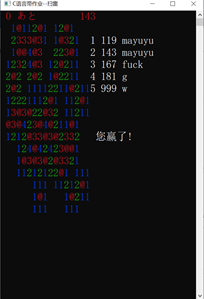## 调试时的一些注意事项

此程序使用了控制台编程中的鼠标操作函数，该函数在windows7及以下系统能正常工作，windows10系统需要在兼容模式下运行，且需关掉快速编辑模式
操作方法如下：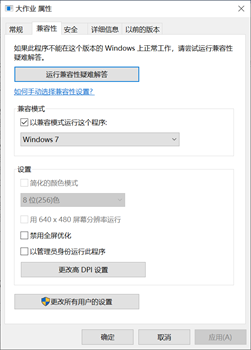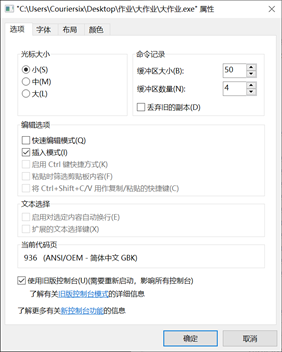## 最后附上完整的代码

#include<bits/stdc++.h>
#include <Windows.h>
using namespace std;
int n,m,t,mode,d=11;
char id; //用户进入游戏时要给自己起个名字
bool f=false,start=false; //start:游戏是否开始
int s,e,duration=999;
HANDLE hin=NULL;
HANDLE hout=NULL;
HANDLE houtput=NULL;
int dx={0,0,1,-1,1,-1,1,-1};
int dy={1,-1,0,0,-1,1,1,-1};
int rank;  //记录用时排名
char name; //rating表中每一个成员的名字
struct {
bool occupy=true,flag=false,vis=false; //occupy:此处有数字或者地雷或者已被探索  flag:此处插了棋子
char ch;   //此处存的内容
int num=0;
}mapp;
void gotoxy(int x, int y)  //光标移动函数
{
COORD pos1= {x, y};
SetConsoleCursorPosition(houtput, pos1);
}
void write(int k){ //彩色字符输出函数1-8，11和-11是处理旗子的情况
WORD w1;
switch (k){
case 0:w1=0x0f; break;
case 1:w1=0x01; break;
case 2:w1=0x02; break;
case 3:w1=0x04; break;
case 4:w1=0x01; break;
case 5:w1=0x04; break;
case 6:w1=0x06; break;
case 7:w1=0x06; break;
case 8:w1=0x0f; break;
case -11:w1=0x04; break;
case 11:w1=0x0f; break;
}
SetConsoleTextAttribute(houtput,w1);
if (k>=1 && k<=8)
cout<<k;
else{
if (k==0) cout<<' ';
if (k==-11) cout<<'@';
if (k==11) cout<<'#';
}
}
void qsort(int m,int l,int r){
int i,j,mid,tmp;
char tmp1;
i=l; j=r; mid=rank[m][(i+j)/2];
while (true){
while (rank[m][i]<mid) i++;
while (rank[m][j]>mid) j--;
if (i<=j){
tmp=rank[m][i]; rank[m][i]=rank[m][j]; rank[m][j]=tmp;
for (int k=0; k<100; k++){
tmp1=name[5*(m-1)+i][k]; name[5*(m-1)+i][k]=name[5*(m-1)+j][k]; name[5*(m-1)+j][k]=tmp1;
}

i++; j--;
}
if (i>j) break;
}
if (l<j) qsort(m,l,j);
if (i<r) qsort(m,i,r);
}
void rating(){
int x; //无实际意义，只是为了读文件过滤数字
FILE *fp1,*fp2;
fp1=freopen("data.txt","r",stdin);
for (int i=1; i<=3; i++)
for (int j=1; j<=5; j++){
cin>>x>>rank[i][j]; gets(name[5*(i-1)+j]);
}

fclose(fp1);
if (duration<=rank[mode]){
rank[mode]=duration;
for (int i=0; i<10; i++){
name[5*(mode-1)+5][i]=id[i];
}
qsort(mode,1,5);
}
gotoxy(n+1,2);
for (int j=1; j<=5; j++){  //将排行榜打在公屏上
gotoxy(n+1,1+j);
cout<<j<<' '<<rank[mode][j]<<' '; puts(name[5*(mode-1)+j]);
}

fp2=freopen("data.txt","w",stdout);
for (int i=1; i<=3; i++)
for (int j=1; j<=5; j++){
cout<<j<<' '<<rank[i][j]; puts(name[5*(i-1)+j]);
}

fclose(fp2);
}
int check(){   //判断是否游戏结束
if (f) return 0; //踩了雷，bad end
for (int i=1; i<=n; i++)
for (int j=1; j<=m; j++)
if (mapp[i][j].occupy)
return 1;
return 0;
}
void dfs(int x,int y){
int x1,y1;
gotoxy(x,y); write(mapp[x][y].num); mapp[x][y].occupy=false; mapp[x][y].vis=true;
if (mapp[x][y].num>0) return;  //扩展到数字时停止搜索
for (int i=0; i<7; i++){
x1=x+dx[i]; y1=y+dy[i];
if (!mapp[x1][y1].vis &&  x1>0 && y1>0 && x1<=n && y1<=m){
dfs(x1,y1);
}
}
}
INPUT_RECORD mouseRecord;
DWORD res;
COORD pos;
WORD wr1;
hin = GetStdHandle(STD_INPUT_HANDLE);  //标准句柄输入
hout = GetStdHandle(STD_OUTPUT_HANDLE); //标准句柄输出
while (1)
{
pos = mouseRecord.Event.MouseEvent.dwMousePosition; //获取当前鼠标位置
if (mouseRecord.EventType == MOUSE_EVENT)
{
if (mouseRecord.Event.MouseEvent.dwButtonState == FROM_LEFT_1ST_BUTTON_PRESSED) //按下左键时开雷
{
if (!mapp[(int)pos.X][(int)pos.Y].vis && (int)pos.X>0 && (int)pos.X<=n && (int)pos.Y>0 && (int)pos.Y<=m && !mapp[(int)pos.X][(int)pos.Y].flag){
//该处未被访问且为合法坐标
if (mapp[(int)pos.X][(int)pos.Y].ch=='*' && !mapp[(int)pos.X][(int)pos.Y].flag){
wr1=0x04; SetConsoleTextAttribute(houtput,wr1);
gotoxy(n+2,6); cout<<"您输了!";
gotoxy((int)pos.X,(int)pos.Y); cout<<'*'; f=true;
Sleep(5000);
break;
}
else{
start=true;
dfs((int)pos.X,(int)pos.Y); //使用深度优先搜索扩展空白区域
if (!check()){
wr1=0x0f; SetConsoleTextAttribute(houtput,wr1);
gotoxy(n+2,10); cout<<"您赢了!";
rating(); //排行榜制作
Sleep(5000);
break;
}
}
}
}
if (mouseRecord.Event.MouseEvent.dwButtonState == RIGHTMOST_BUTTON_PRESSED) //按下右键时插旗子/拔旗子
{
if (!mapp[(int)pos.X][(int)pos.Y].vis && (int)pos.X>0 && (int)pos.X<=n && (int)pos.Y>0 && (int)pos.Y<=m){
//该位置是合法坐标且未被访问过，实际演示中表现为只能在#上插旗子
gotoxy((int)pos.X,(int)pos.Y);
if (mapp[(int)pos.X][(int)pos.Y].flag){
write(d);
t++;
}
else{
write(-d); t--;
}
SetConsoleTextAttribute(houtput,0x04);
gotoxy(1,0); cout<<"  ";
gotoxy(1,0); cout<<t;
mapp[(int)pos.X][(int)pos.Y].flag=!mapp[(int)pos.X][(int)pos.Y].flag;
}
}
//if (mouseRecord.Event.MouseEvent.dwEventFlags==MOUSE_MOVED)
//{
e=clock();
duration=(int)(e-s) / CLOCKS_PER_SEC;
SetConsoleTextAttribute(houtput,0x04);
gotoxy(n-1,0);
if (duration<=999) printf("%d",duration);
//}
}
}
//关闭句柄
CloseHandle(hin);
CloseHandle(hout);
}
void initialization(){  //初始化游戏界面
int len,width,x1=0,y1=0;
//houtput=GetStdHandle(STD_OUTPUT_HANDLE);
SetConsoleTitleA("C语言带作业--扫雷");
cout<<"请先给自己起个名字";
gotoxy(0,1);
gets(id);
gotoxy(0,0);
cout<<"请选择要玩的模式\n";
cout<<"1:初级\n";
cout<<"2:中级\n";
cout<<"3:高级\n";
cout<<"4:自定义\n";
gotoxy(0,5);
cin>>mode;
for (int i=1; i<=10; i++){
gotoxy(0,i-1);
cout<<"                                ";
}
s=clock();
switch (mode){
case 1:{n=8; m=8; t=10; len=28; width=28; break;}  //初级： 8x8 10个雷
case 2:{n=16; m=16; t=40; len=36; width=36; break;} //中级：16x16 40个雷
case 3:{n=30; m=16; t=99; len=50; width=36; break;} //高级： 30x16 99个雷
case 4:{gotoxy(0,0);
for (int i=1; i<=6; i++){
cout<<"                 \n";
}
gotoxy(0,0);
scanf("%d%d%d",&n,&m,&t); len=n+20; width=m+20;
break;
}
//自定义棋盘大小和雷数
}
WORD wr1 =0x0f;//定义颜色属性；第一位为背景色，第二位为前景色
SetConsoleTextAttribute(houtput,wr1);
srand(time(NULL)); //防伪随机
int ok;
for (int i=1; i<=t; i++){  //使用随机函数rand()%(y-x)+x，枚举棋盘范围内的所有地雷的随机位置坐标
do{
x1=rand()%(n-1)+1; y1=rand()%(m-1)+1; ok=1;
if (mapp[x1][y1].occupy){
mapp[x1][y1].occupy=false; mapp[x1][y1].ch='*'; ok=0;
}
}while(ok);
}
gotoxy(0,0);
cout<<"                              ";
SetConsoleTextAttribute(houtput,0x04);
gotoxy(1,0); cout<<t<<"あと"; //gotoxy(n/2-1,0); cout<<;
for (int i=1; i<=5; i++){
gotoxy(0,i); putchar(' ');
}
SetConsoleTextAttribute(houtput,0x0f);
for (int i=1; i<=n; i++){
for (int j=1; j<=m; j++){
gotoxy(i,j); putchar('#');
for (int k=0; k<8; k++)
if (mapp[i+dx[k]][j+dy[k]].ch=='*' && mapp[i][j].occupy)
mapp[i][j].num++;
}
}
}
int main(){
houtput=GetStdHandle(STD_OUTPUT_HANDLE);
initialization();
return 0;
}

展开全文c++
• 本次C语言实验主要包括三个实验，分别是：一、摄氏温度C与华氏温度F转换并打印对照表；二、输出螺旋矩阵ｎ＊ｎ（２≤ｎ≤９）；三、用二维数组实现三角函数在以°为单位的区间［０，ａｎｇ）图像。
• 一下，我们的C语言课程布置了一次大作业，要求做出一个管理系统，整个系统可以设计为数据插入模块、数据修改模块、数据删除模块、数据显示模块和数据查询模块。各种信息管理系统本质上是没有太区别的，对于...

# 图书管理系统

## 1.前言

在大一下，我们的C语言课程布置了一次大作业，要求做出一个管理系统，整个系统可以设计为数据插入模块、数据修改模块、数据删除模块、数据显示模块和数据查询模块。各种信息管理系统本质上是没有太大区别的，对于数据的操作都是差不多的。

## 2.功能及其相关函数

### 2.1 菜单界面的构建

建立两个界面函数，用来搭建主页,这里用到两个头文件
#include<conio.h>
定义了通过控制台的数据输入与输出函数
#include<windows.h>
用来调整方框大小与背景颜色

#include<conio.h>
#include<windows.h>

void Menu1()
{

system("mode con cols=54 lines=30");
system("color F2");
printf("**********欢迎使用****大学图书管理系统***********\n");

}//标题函数1

{
printf("~~~~~~~~~~~~~~~~~~~~~~~~~~~~~~~~~~~~~~~~~~~~~~~~~~~~~\n");
printf("\t\t1.录入图书\t\t\n");
printf("\t\t2.显示图书\t\t\n");
printf("\t\t3.查询图书\t\t\n");
printf("\t\t4.修改图书\t\t\n");
printf("\t\t5.删除图书\t\t\n");
printf("\t\t0.退出\t\t\n");
printf("\t\t输入你的操作：");

}//标题函数2


### 2.2 功能一 录入图书

设置一个WriteToFile()函数，利用scanf函数将数据输入给结构体，利用fwrite函数将结构体数据写入"book1.dat"这个二进制文件中。利用while函数可以进行反复录入。

### 2.3 功能二 显示图书

while (!feof(fp))
{
{
printf("%10s\t%8s\t\t%5s\n", stu.id, stu.name, stu.author);
}
}


此时利用if语句可以有效避免结构体数据被重复读出。

### 2.5 功能四 修改图书

此时利用strcmp函数进行图书ID的“检索”,并将其结构体内的所有数据进行重新输入，达到修改的目的。此时的fseek函数有确定文件指针的效果。

### 2.6 功能五 删除图书

删除图书原理：重新建立一个临时文件，将除了需要旧文件删除的内容全部复制到临时文件中，再利用remove函数删除掉旧文件，使用rename函数将临时文件改名为旧文件名字。达到删除的目的

## 3.注意事项

（1）：可以利用链表简化程序
（2）：应该要尽可能
扩充管理系统的功能
（3）：本人使用VS2017编写程序，不同的版本可能不兼容
（4）：main()中利用到do-while和switch函数实现主页菜单的效果# 4.源程序

#define _CRT_SECURE_NO_WARNINGS
#include<stdio.h>
#include<string.h>
#include<stdlib.h>
#include<conio.h>
#include<windows.h>
#define LEN sizeof(book)

typedef struct book //确认结构体
{

char id;
char name;
char author;
char publish;
double price;

}book;

void WriteToFile();//书籍信息输入的函数

void QueryFile();//书籍的查询函数

void ModifyFile();//书籍的修改函数

void DeletFile();//删除数据的函数

int main()
{
int select;

do
{
scanf("%d", &select);
switch (select)
{
case 1:
WriteToFile();
break;

case 2:
break;

case 3:
QueryFile();
break;

case 4:
ModifyFile();
break;

case 5:
DeletFile();
break;

default:
printf("退出程序！");
exit(0);
break;
}
}while ((select == 1 || select == 2)||(select == 3|| select == 4)||( select == 5));

return 0;
}//利用switch函数进行菜单的选择

{

system("mode con cols=54 lines=30");
system("color F2");
printf("**********欢迎使用长沙理工大学图书管理系统***********\n");

}//标题函数1

{
printf("~~~~~~~~~~~~~~~~~~~~~~~~~~~~~~~~~~~~~~~~~~~~~~~~~~~~~\n");
printf("\t\t1.录入图书\t\t\n");
printf("\t\t2.显示图书\t\t\n");
printf("\t\t3.查询图书\t\t\n");
printf("\t\t4.修改图书\t\t\n");
printf("\t\t5.删除图书\t\t\n");
printf("\t\t0.退出\t\t\n");
printf("\t\t输入你的操作：");

}//标题函数2

void WriteToFile()
{
FILE *fp = NULL;
book stu;
char flag = 'y';
fp = fopen("book1.dat", "ab+");//打开文件

if (fp == NULL)
{
printf("文件打开失败！\n");
exit(1);//1表示在有错的方式退出程序
}

while ((flag == 'y' || flag == 'Y'))
{
system("cls");

printf("请输入图书id：");
scanf("%s", stu.id);

printf("请输入书名：");
scanf("%s", stu.name);

printf("请输入书籍作者：");
scanf("%s", &stu.author);

printf("请输入出版社：");
scanf("%s", &stu.publish);

printf("请输入价格：");
scanf("%lf", &stu.price);

fwrite(&stu, LEN, 1, fp);
fflush(stdin);

printf("继续输入吗？继续请输入y或Y：");
getchar();
scanf("%c", &flag);
}

fclose(fp);//关闭文件
return;
}//图书添加的函数

{
system("cls");
FILE *fp = NULL;
book stu;
fp = fopen("book1.dat", "rb");

if (fp == NULL)
{
printf("文件打开失败");
exit(1);
}
printf("~~~~~~~~~~~~~~~~~~~~~~~~~~~~~~~~~~~~~~~~~~~~~~~~~~~~~\n");
printf("         id\t\t书名\t\t作者\n");

fseek(fp, 0, SEEK_SET);

while (!feof(fp))
{
{
printf("%10s\t%8s\t\t%5s\n", stu.id, stu.name, stu.author);
}
}

printf("~~~~~~~~~~~~~~~~~~~~~~~~~~~~~~~~~~~~~~~~~~~~~~~~~~~~~\n");
system("pause");
system("cls");
fclose(fp);
return;
}

void QueryFile()
{
system("cls");
book stu;
char x;
int flag = 0;
FILE *fp;

printf("~~~~~~~~~~~~~~~~~~~~~~~~~~~~~~~~~~~~~~~~~~~~~~~~~~~~~\n");
printf("\t\t请输入图书id:");
scanf("%s", x);
printf("  ID  书名    作者     出版社     价格\n");

fp = fopen("book1.dat", "rb");

if (fp == NULL)
{
printf("~~~~~~~~~~~~~~~~~~~~~~~~~~~~~~~~~~~~~~~~~~~~~~~~~~~~~\n");
printf("错误\n");
printf("~~~~~~~~~~~~~~~~~~~~~~~~~~~~~~~~~~~~~~~~~~~~~~~~~~~~~\n");
return;
}

fseek(fp, 0, SEEK_SET);
{

if (strcmp(x, stu.id) == 0)
{
printf("%3s  %5s  %5s    %10s %5.2lf\n", stu.id, stu.name, stu.author, stu.publish, stu.price);
flag = 1;
}

if (flag = 0)
{
printf("~~~~~~~~~~~~~~~~~~~~~~~~~~~~~~~~~~~~~~~~~~~~~~~~~~~~~\n");
printf("没有图书信息");
printf("~~~~~~~~~~~~~~~~~~~~~~~~~~~~~~~~~~~~~~~~~~~~~~~~~~~~~\n");
}
}

printf("~~~~~~~~~~~~~~~~~~~~~~~~~~~~~~~~~~~~~~~~~~~~~~~~~~~~~\n");
fclose(fp);
system("pause");
system("cls");
return;
}

void ModifyFile()
{
system("cls");
book stu;
FILE *fp;
char x;

printf("~~~~~~~~~~~~~~~~~~~~~~~~~~~~~~~~~~~~~~~~~~~~~~~~~~~~~\n");
printf("请输入图书id:");
scanf("%s", x);

fp = fopen("book1.dat", "rb+");

if (fp == NULL)
{
printf("文件打开失败");
exit(1);
}

fseek(fp, 0, SEEK_SET);
{

if (strcmp(x, stu.id) == 0)
{
printf("请重新输入图书id:   ");
scanf("%s", stu.id);

printf("请重新输入书名:    ");
scanf("%s", stu.name);

printf("请重新输入书籍作者  : ");
scanf("%s", &stu.author);

printf("请重新输入图书出版社  : ");
scanf("%s", &stu.publish);

printf("请重新输入图书价格 :   ");
scanf("%lf", &stu.price);
printf("~~~~~~~~~~~~~~~~~~~~~~~~~~~~~~~~~~~~~~~~~~~~~~~~~~~~~\n");
fflush(stdin);
fseek(fp, 0-LEN, SEEK_CUR);
fwrite(&stu, LEN, 1, fp);
fclose(fp);
}

if (feof(fp))
{
printf("~~~~~~~~~~~~~~~~~~~~~~~~~~~~~~~~~~~~~~~~~~~~~~~~~~~~~\n");
printf("没有图书信息");
printf("~~~~~~~~~~~~~~~~~~~~~~~~~~~~~~~~~~~~~~~~~~~~~~~~~~~~~\n");
}

}

system("pause");
system("cls");
return;
}

void DeletFile()
{
system("cls");
book s;
FILE* fp;
char a;
fp = fopen("book1.dat", "rb+");

if (fp == NULL)
{
printf("打开文件错误！！！\n");
exit(1);
}

printf("\n请输入图书ID：");
scanf("%s", a);
printf("\n\t\t\t删除成功\n");

fseek(fp, 0, SEEK_SET);
FILE* fp1;
fp1 = fopen("linshi.dat", "ab+");//读写新建一个临时文件

{
if (strcmp(a, s.id) != 0)//不是要删除的内容
{
fwrite(&s, LEN, 1, fp1);
}
}

fclose(fp);
fclose(fp1);
remove("book1.dat");//删除原文件
rename("linshi.dat", "book1.dat");//重命名为原文件

fflush(stdin);
system("pause");
system("cls");
return;
}


展开全文昆明理工大学大一C语言大作业题目

展开全文• 6.按学号由小到排出成绩表 7.按姓名的字典顺序排出成绩表 8.按学号查询学生排名及其考试成绩 9.按姓名查询学生排名及其考试成绩 10.按优秀（90-100）、良好（80-89）、中等（70-79）、及格（60-69）、不及格...
• #include#include#includestruct A {// 结构体Achar c;//数据};struct B { //结构体Bint number;//链表节点号struct A a; //包含结构体Astruct B* next;//形成链表};//链表头尾指针struct B* R_head = NULL;...
• // 计算所有学生的平均分 } } void sort(int l, int r){// 这里用了快排排序将平均分按从到小排列（处理的数据更多） if(l >= r) return ; int i = l, j = r; struct student key = stu[i]; while(i ){ while(i [j...
• #include <stdio.h> #include <stdlib.h> #include <math.h> #include <string.h> int over = 1; int change = 1;...// 画布由20行，36列的二维数组成，通过符号常数定义 ...#def
• 大一写的结课作业，代码用到了指针、文件、数组、结构体、函数，包括增删改查、保存信息到文件和成绩排名等功能，欢迎大家下载使用。
• C语言大学课程作业 含课程报告 自我撰写 无抄袭无参考 满分 400行代码
• ## 大一c语言实验报告

千次阅读 2021-05-18 10:15:53
篇一：大一上期C语言实验报告3 基本输入输出成都工业学院·计算机工程学院 《程序设计基础》实验报告1. 实验目的(1) 熟练掌握scanf()/printf()库函数的调用格式，正确使用各种格式转换控制符，掌握各种类型数据的...
• 大一写的结课作业，代码用到了指针、文件、数组、结构体、函数，包括登录、注册、增删改查等功能，欢迎大家下载使用。
• 我是2014级计算机与信息技术学院的学生，一年下来我也想为自己留下些东西，同时希望...其中包括我的C语言上机题目、上机代码、上机总结，希望以后的学弟学妹能有所借鉴，能更快的完成作业，不要认为C语言难学，努力！
• 大一c语言习题练习与答案程序设计与C语言1.1 选择题考点：标识符的命名规则只能由字母、数字、下划线构成数字不能作为标识符的开头关键字不能作为标识符选项A中的“-” ，选项B中“[”与“]”不满足(1)；...
• 请编写一个程序，颠倒一个无符号整数的二进制位的顺序。该程序请用户输入一个整数值，然后调用reverseBits来打印其反序的二进制位。按二进制格式打印该整数颠倒处理前后的值，以验证数位已被正确的颠倒了。...
• c语言期末大作业，信息管理系统，包含如下知识点：结构体、数组、文件操作等等，包含了c语言中几乎所有知识点，具有清屏效果
• C语言大作业—森林冰火人大作业简介第三方库界面图片切换冰火娃动作图片切换冰娃火娃的跑动地面，墙壁，冰水，火水与毒液等的判断代码实现头文件变量简介结构体及数据初始化冰火娃图片切换冰火娃动作出口&...
• 课本上的程序，一般没什么用，想上这个网站找找自己需要的东西
• C语言模拟通讯录（大一C语言期末大作业）.doc
• C语言初学者的课程设计，适用于大一新生，方便好用。包括实验报告和源代码。...